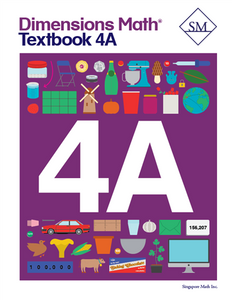# Dimensions Math Textbook 4A

Vendor
Singapore Math Inc.
Regular price
\$13.70
Sale price
\$13.70
Quantity must be 1 or more

The Dimensions Math® PK-5 series is a new curriculum designed by Singapore Math Inc. to better serve U.S. teachers and students. Aspects of Singapore math curriculum have been updated for clarity and relevance, while preserving the solid foundation that makes it unique. The series follows the principles outlined in the Singapore Mathematics Framework and uses the Concrete > Pictorial > Abstract approach. The curriculum also incorporates Singapore mental math strategies and model drawing.

In the Dimensions Math program, concepts are introduced and explained in a new format with vibrant imagery. The implementation of this curriculum suits today’s needs, while the progression and scope that define Singapore math remain intact.

The Dimensions Math 4A Textbook corresponds to the 4A workbook (required and sold-separately). This textbook also features hands-on workpage elements alongside very simple and direct lessons. Concepts are taught through immersive, visual scenarios created with five characters: Emma, Alex, Sofia, Dio, and Mei. Logically sequenced lessons provide a strong foundation for increasingly complicated math concepts. Chapters in 4A cover numbers to one million, addition and subtraction, multiples and factors, multiplication, division, fractions, adding and subtracting fractions, multiplying a fraction and a whole number, and line graphs and line plots.